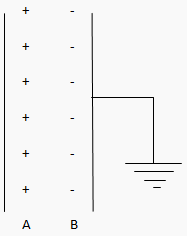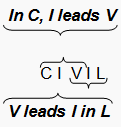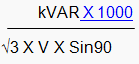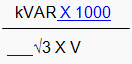top of page

Search

# Capacitor Banks in Power System

Updated: Feb 2, 2022

### Capacitance

When a charge is delivered to a conductor its potential is raised in proportion to the quantity of charge given to it.At a particular potential, a conductor can hold a given amount of charge. Capacitance is the term to indicate the limited ability to hold charge by a conductor.

• Let charge given to a conductor be = q

• Let V be the potential to which it is raised.

Then q α V, or

q = CV

C is constant for a conductor depending upon its shape size and the surrounding medium. This constant is called the capacitance of a conductor.

If V = 1 Volt then C = Q, thus capacitance is defined as the amount of electric charge in coulomb required to raise its potential by one volt.

If V = 1 Volt then C = Q, and Q = 1 Coulomb then C = 1 Farad thus one Farad is the capacitance of a capacitor which stores a charge of one coulomb when a voltage of one volt is applied across its terminal### Capacitor

A capacitor or condenser is a device for storing a large quantity of electric charge. Though the capacity of a conductor to hold a charge at a particular potential is limited, it can be increased artificially. Thus any arrangement for increasing the capacity of a conductor artificially is called a capacitor.

• Capacitors are of many types depending upon its shape, like parallel plate, spherical and cylindrical capacitors etc….

• In a capacitor, there are two conductors with equal and opposite charges say +q and –q. Thus q is called the charge of the capacitor and the potential difference is called the potential of the capacitor.If a second conductor B is kept closed to A then electrostatic induction takes place. –q units of charge are induced on the nearer face of B and +q units of charge is induced on the farther face of B. Since B is earthed the charge +q will be neutralized by the flow of electrons from the earth.

Potential of A due to self-charge = V

Potential of A due to –q charge on B = -V’

Thus the net potential of A = V + (-V’) = V -V’ which is less than V

Hence the potential of A has been decreased keeping the charge on it fixed, hence capacitance has been increased

With the presence of B, the amount of work done in bringing a unit positive charge from infinity to conductor A decreases as there will be a force of repulsion due to A and attraction due to B. Thus the resultant force of repulsion is reduced on unit positive charge and consequently, the amount of work done is less and finally due to this potential of A decreases

Therefore the capacity of A to hold a charge (Capacitance) is increased.

### Dielectric Strength

The material between the two conductors A and B as shown in the figure above is always some dielectric material. Under normal operating conditions the dielectric materials have very few free electrons. If the electric field strength between a pair of charged plates is gradually increased, some of the electrons may be detached from the dielectric resulting in a small current.

When the electric field strength applied to a dielectric exceeds a critical value, the insulating properties of the dielectric material gets destroys and starts conducting between the two conductors A and B.

This is called a breakdown of the dielectric which is a fault condition for a capacitor bank. The minimum potential gradient required to cause such a breakdown is called the dielectric strength of the material. It measures the ability of a dielectric to withstand breakdown. It is expressed as kV/mm.

It is reduced by moisture, high temperature; ageing etc. The below table gives the dielectric strength of some dielectricsDielectric Strength for the capacitor is the maximum peak voltage that the capacitor is rated to withstand at room temperature. Test by applying the specified multiple of rated voltage for one minute through a current limiting resistance of 100 Ω per volt.

### Sizing of Capacitor banks for power factor improvement

The Power Factor Correction of electrical loads is a problem common to all industrial companies. Every user which utilizes electrical power to obtain work in various forms continuously asks the mains to supply a certain quantity of active power together with reactive power

Most loads on an electrical distribution system can be placed in one of three categories:

• Resistive

• Inductive

• Capacitive

The most common of these on modern systems is the inductive load. Typical examples include a transformer, fluorescent lighting, AC induction motors, Arc/induction, furnaces etc. which draw not, only active power from the supply, but also inductive reactive power (kVAr). Common characteristics of these inductive loads are that they utilize a winding to produce an electromagnetic field which allows the motor or transformer to function and requires a certain amount of electrical power in order to maintain the field

''Therefore Active Power (KW) actually performs the work whereas Reactive Power (KVAr) sustains the electromagnetic field. This reactive power though is necessary for the equipment to operate correctly but could be interpreted as an undesirable burden on the supply''

If we quantify the power factor improvement aspect from the utility company’s point of view then raising the average operating power factor of the network from 0.7 to 0.9 means:

• Cutting costs due to ohmic losses in the network by 40%

• Increasing the potential of production and distribution plants by 30%.

These figures speak for themselves: it means saving hundreds of thousands of tons of fuel and making several power plants and hundreds of transformer rooms available.

Thus in the case of low power factors, utility companies charge higher rates in order to cover the additional costs they must incur due to the inefficiency of the system that taps energy. It is a well-known fact that electricity users relying on an alternating current – with the exception of heating elements – absorb from the network not only the active energy they convert into mechanical work, light, heat, etc. but also inductive reactive energy whose main function is to activate the magnetic fields necessary for the functioning of electric machines.

Power Factor is also defined as cos Ø = kW / KVA

One can see after the compensation requirement of kVAR (equal to kVAR1 – kVAR2) from the system has gone down.

Since kVA = kW + kVAR decreased kVAR requirement from the system has will result in decreased kVA requirement, which will consequently result in lower current consumption from the source.

Point to be noted in this case that any load which was operating at a power factor of 0.85 before compensation continues to operate on the same power factor of 0.85 even after compensation. It is the source power factor that has been improved by compensating the kVAR requirement of that particular load (or group of loads) from parallel-connected capacitor banks. The source is now relieved of providing some amount of kVAR (=kVAR1 – kVAR2).

COMPENSATED kVAR = kVAR1 – kVAR2 = kW tanØ1 – tan Ø2 = kW [tanØ1 – tan Ø2]Power Factor Triangle

Hence Required Rating of Capacitor banks to be connected = kW [tanØ1 – tan Ø2]

Where,

cos Ø1 = Operating Power Factor cos Ø2 = Target Power Factor or Power Factor after improvementAutomatic capacitor banks consist of stages controlled by a power factor controller which ensures that the required capacitor power is always connected to the system, which means that always would be an optimal correction (photo credit: energolukss.lv)

### Sizing of switching device for Capacitor banks

It should be noted that in an inductance the current lags the voltage by 90 degrees and in a capacitor, the current leads the voltage by 90 degrees. These relationships are very important for drawing phasor diagrams.

It is very convenient to remember these relationships by the word CIVIL as follows:Hence Current drawn from Capacitor bank =Since sin90 = 1 hence the equation for current drawn can be rewritten as:The relevant Standards on this device recommend a continuous overload capacity of 30%. A capacitor can have a tolerance of up to +15% in its capacitance value. All current-carrying components such as breakers, contactors, switches, fuses, cables and busbar systems associated with a capacitor unit or its banks, must therefore be rated for at least 1.5 times the rated current

The rating of a capacitor unit will thus vary in a square proportion of the effective harmonic voltage and in a direct proportion to the harmonic frequency. This rise in kVAR, however, will not contribute to the improvement of the system power factor. but only of the overloading of the capacitors themselves

Therefore it may, however, sometimes be desirable to further enhance the overloading capacity of the capacitor and so also the rating of the current-carrying components if the circuit conditions and type of loads connected on the system are prone to generate excessive harmonics.

Examples are when they are connected to a system on which we operate static drive and arc furnaces. It is desirable to contain the harmonic effects as far as practicable to protect the capacitors as well as inductive loads connected on the system and the communication network if running in the vicinity.

1. Hence as per the above discussion when determining the actual load current of a capacitor unit in operation, a factor of 1.15 is additionally considered to account for the allowable tolerance in the capacitance value of the capacitor unit.

2. Effective kVAR = 1.3 x 1. I5 = 1.5 times the rated kVAR and for which all switching and protective devices must be selected

### Taking care of harmonics

It is common practice to leave the star-connected capacitor banks ungrounded when used in the system or use delta-connected banks to prevent the flow of third harmonic currents into the power system through the grounded neutral.

Use of filter circuits in the power lines at suitable locations, to drain the excessive harmonic quantities of the system into the filter circuits.

A filter circuit is a combination of capacitor and series reactance, tuned to a particular harmonic frequency (series resonance), to offer it the least impedance at that frequency and hence, filter it out.

Say, for the fifth harmonic, Xc5 = XLS

The use of a reactor in series with the capacitors will reduce the harmonic effects in a power network, as well as their effect on other circuits in the vicinity, such as a telecommunication network. The choice of reactance should be such that it will provide the required detuning by resonating below the required harmonic, to provide a least impedance path for that harmonic and filter it out from the circuit.

The basic idea of a filter circuit is to make it respond to the current of one frequency and reject all other frequency components. At power frequency, the circuit should act as a capacitive load and improve the p.f. of the system.

For the fifth harmonic, for instance, it should resonate below 5 x 50 Hz for a 50 Hz system, say at around 200-220 Hz, to avoid excessive charging voltages which may lead to:

• Overvoltage may saturate transformer cores and

• Failure of capacitor units and inductive loads connected generate harmonics in the system

It should be ensured that under no condition of system disturbance would the filter circuit become capacitive when it approaches near resonance. To achieve this, the filter circuits may be tuned to a little less than the defined harmonic frequency

Doing so will make the Land hence XL, always higher than Xc, since This provision will also account for any diminishing variation in C, as may be caused by ambient temperature, production tolerances or failure of a few capacitor elements or even of a few units during operation.

The power factor correction system would thus become inductive for most of the current harmonics produced by power electronic circuits and would not magnify the harmonic effects or cause disturbance to a communication system if existing in the vicinity A filter circuit can be tuned to the lowest (say the fifth) harmonic produced by an electronic circuit. This is because LT capacitors are normally connected in delta and hence do not allow the third harmonic to enter the circuit while the HT capacitors are connected in star, but their neutral is left floating and hence it does not allow the third harmonic to enter the circuit

In non-linear or unbalanced loads, however, the third harmonic may still exist. For a closer compensation, uni-frequency filters can be used to compensate individual harmonic contents by tuning the circuit to different harmonics

For more exact compensation, the contents and amplitudes of the harmonic quantities present in the system can be measured with the help of an oscilloscope or a harmonic analyzer before deciding on the most appropriate filter circuit/circuits. Theoretically, a filter is required for each harmonic, but in practice, filters adjusted for one or two lower frequencies are adequate to suppress all higher harmonics to a large extent and save on cost.

If we can provide a series reactor of 6% of the total kVAR of the capacitor banks connected on the system, most of the harmonics present in the system can be suppressed. With this reactance, the system would be tuned to below the fifth harmonic (at 204 Hz) for a 50Hz system.

### Working of APFC Relay

The basic principle of this relay is the sensing of the phase displacement between the fundamental waveforms of the voltage and current waves of the power circuit. Harmonic quantities are filtered out when present in the system. This is a universal practice to measure the p.f. of a system to economize on the cost of the relay. The actual p.f. of the circuit may therefore be less than measured by the relay.

But one can set the relay slightly higher (less than unity), to account for the harmonics, when harmonics are present in the system. From this phase displacement, a D.C. voltage output is produced by a transducer circuit.

The value of the D.C. voltage depends upon the phase displacement, i.e. the p.f. of the circuit. This D.C. voltage is compared with a built-in reference D.C. voltage, adjustable by the p.f. a setting knob or by selecting the operating band provided on the front panel of the relay. Corrective signals are produced by the relay to switch ON or OFF the stage capacitors through a built-in sequencing circuit to reach the desired level of p.f. A little lower p.f. then set would attempt to switch another unit or bank of capacitors, which may overcorrect the set p.f.

Now the relay would switch off a few capacitor units or banks to readjust the p.f. and so will commence a process of hunting, which is undesirable. To avoid such a situation the sensitivity of the comparator is made adjustable through the knob on the front panel of the relay.

The sensitivity control can be built in terms of phase angle (normally adjustable from 4 to 14 degrees electrical) or percentage kVAR. The sensitivity, in terms of an operating band, helps the relay to avoid a marginal overcorrection or under correction and hence the hunting.

As soon as the system’s actual p.f. deviates from the pre-set limits, the relay becomes activated and switches in or switch out capacitor units one by one, until the corrected p.f. falls within the sensitivity limit of the relay.

The power factor correction relays are normally available in three versions:

1. Electromagnetic (being quickly outdated). They are very slow and may take up to 2 minutes or more to initiate a correction.

2. Solid state-based on discrete ICs.

3. Solid state-based on micro-controllers (microprocessors).

A time delay is built in to allow the discharge of a charged capacitor up to 90% before it is switched. This is achieved by introducing a timer into the relay’s switching circuit. The timer comes on whenever an OFF signal occurs, and blocks the next operation of a charged capacitor, even on an ON command, until it is discharged to at least 90% of the applied voltage. This feature ensures safety against overvoltage.

Normally this time is 1-3 minutes for LT and 5-10 minutes for HT shunt capacitors unless fast-discharge devices are provided across the capacitor terminals to reduce this time. Fast-discharge devices are sometimes introduced to discharge them faster than these stipulations to match with quickly varying loads.

The ON action begins only when the timer is released. The time of switching between each relay step is, however, quite short, of the order of 3-5 seconds. It includes the timings of the control circuit auxiliary relays (contactors). It may be noted that of this, the operating time of the static relay is scarce of the order of three to five cycles.

In rapidly changing loads it must be ensured that enough discharged capacitors are available in the circuit on every close command. To achieve this, sometimes it may be necessary to provide special discharge devices across the capacitor terminals or a few extra capacitor units to keep them ready for the next switching. It may require a systematic study on the pattern of load variations and the corresponding p.f. Fast switching, however, is found more often in LT systems than in HT. HT systems are more stable, as the variable loads are mostly LT.

The above discussion is generally related to IC-based solid-state relays and in most parts to microprocessor-based relays of the more rudimentary types.

### Power Factor Correction of Induction Motor

The selection of capacitor rating, for an induction motor, running at different loads at different times, due either to change in load or to fluctuation in supply voltage, is difficult and should be done with care because the reactive loading of the motor also fluctuates accordingly.

A capacitor with a higher value of kVAR than the motor kVAR, under certain load conditions, may develop dangerous voltages due to self-excitation.

At the unity power factor, the residual voltage of a capacitor is equal to the system voltage. It rises at leading power factors. These voltages will appear across the capacitor banks when they are switched off and become a potential source of danger to the motor and the operator.

Such a situation may arise when the capacitor unit is connected across the motor terminals and is switched with it. This may happen during an open transient condition while changing over from star to delta, or from one step to another, as in an A/T switching, or during tripping of the motor or even while switching off a running motor.

In all such cases the capacitor will be fully charged and its excitation voltage, the magnitude of which depends upon the p.f. of the system, will appear across the motor terminals or any other appliances connected on the same circuit. The motor, after disconnection from the supply, will receive the self-excitation voltage from the capacitor and while running may act as a generator, giving rise to voltages at the motor terminals considerably higher than the system voltage itself.

The solution to this problem is to select a capacitor with its capacitive current slightly less than the magnetizing current, Im, of the motor, say, 90% of it.

If these facts are not borne in mind when selecting the capacitor rating, particularly when the p.f. of the motor is assumed to be lower than the rated p.f. at full load, then at certain loads and voltages it is possible that the capacitor kVAR may exceed the motor reactive component, and cause a leading power factor. A leading p.f. can produce dangerous overvoltages. This phenomenon is also true in an alternator. If such a situation arises with a motor or an alternator, it is possible that it may cause excessive torques.

Keeping these parameters in mind, motor manufacturers have recommended compensation of only 90% of the no-load kVAR of the motor. irrespective of the motor loading. This for all practical purposes and at all loads will improve the p.f. of the motor to around 0.9-0.95. which is satisfactory. Motor manufacturers suggest the likely capacitor ratings for different motor ratings and speeds

Destek olmak için bana bir kahve ısmarlayabilirsiniz :) ve E-Posta Bültenimize de üye olabilirsiniz…